Courses

# Doc: Mole Fraction Notes | EduRev

## Class 12 : Doc: Mole Fraction Notes | EduRev

The document Doc: Mole Fraction Notes | EduRev is a part of the Class 12 Course Chemistry for JEE.
All you need of Class 12 at this link: Class 12

Mole fraction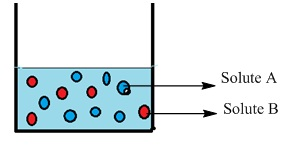Mole fraction can be defined as the ratio of number of moles of the component in the solution to the total number of moles of all components in the solution.

• It is denoted by the alphabet x and subscript written on the right hand side of x denotes the component of which mole fraction is being calculated.
• Mathematically, Mole fraction of a component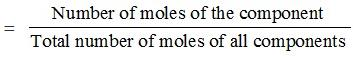• Let us consider a binary mixture of A and B. Let the number of moles of A and B bee nA and nB respectively, then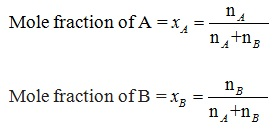• For solution where number of components = i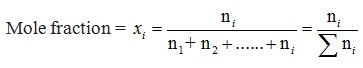• In a given solution sum of the mole fractions of all the components is unity.
Mathematically, x1+ x2+ x3….+ xi = 1

Problem 1. Calculate the mole fraction of benzene in solution containing 30% by mass in carbon tetrachloride.
Solution.
Total mass of the solution = 100 g
Mass of benzene = 30 g.
∴ Mass of carbon tetrachloride = (100 - 30)g = 70 g
Molar mass of benzene (C6H6) = (6 × 12 + 6 × 1) g mol– 1 = 78 g mol-1
∴ Number of moles of C6H6  =30/78 mol
= 0.3846 mol
Molar mass of carbon tetrachloride (CCl4) = 1 × 12 + 4 × 355 = 154 g mol-1
∴ Number of moles of CCl4 = 70/154 mol = 0.4545 mol
Mole fraction of C6H6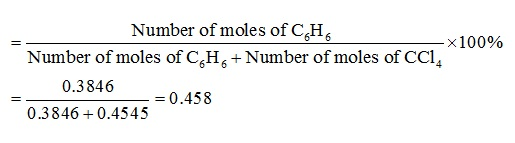Problem 2. A tank is charged with a mixture of 1.0 × 103 mol of oxygen and 4.5 × 103 mol of helium. Calculate the mole fraction of each gas in the mixture.
Solution.
The given parameters are
NHe = 4.5 × 103 mol and NO2 = 1.0 × 103 mol
Mole fraction can be calculated as
XHe = 4.5 × 103 mol / 4.5 × 103 mol + 1.0 × 103 mol
XHe 4.5 mol / 5.5 mol
XHe = 0.82
XO2 = 2.0 × 103 mol / 4.5 × 103 mol + 1.0 × 103 mol
XO2 = 1.0 × 103 / 5.5 × 103
XO2 = 0.18

Problem 3. Determine the mole fraction of methanol CH3OH and water in a solution prepared by dissolving 4.5 g of alcohol in 40 g of H2O. M of H2O is 18 and M of CH3OH is 32.
Solution.
Moles of CH3OH = 4.5 / 32 = 0.14 mole
Moles of H2O = 40 / 18 = 2.2 moles
Therefore, according to the equation
mole fraction of CH3OH = 0.14 / 2.2 + 0.14
= 0.061

Problem 4. What is the mole fraction of carbon tetrachloride (CCl4) in solution if 3.47 moles of CCl4 is dissolved in 8.54 moles of benzene (C6H6)?
Solution.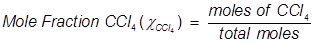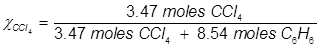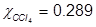Problem 5. What is the mole fraction of formaldehyde (CH2O) in solution if 25.7 grams of CH2O is dissolved in 3.25 moles of carbon tetrachloride (CCl4)?
Solution.
The grams of acetone will need to be converted into moles in order to solve for mole fraction.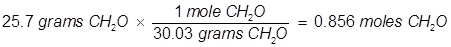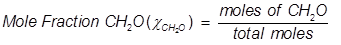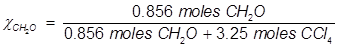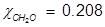Problem 6. 0.100 mole of NaCl is dissolved into 100.0 grams of pure H2O. What is the mole fraction of NaCl?
Solution.
100.0 g / 18.0 g mol-1 = 5.56 mol of H2O
Add that to the 0.100 mol of NaCl = 5.56 + 0.100 = 5.66 mol total
Mole fraction of NaCl = 0.100 mol / 5.66 mol = 0.018
The mole fraction of the H2O:
5.56 mol / 5.66 mol = 0.982

Problem 7. A solution is prepared by mixing 25.0 g of water, H2O, and 25.0 g of ethanol, C2H5OH. Determine the mole fractions of each substance.
Solution.
(1) Determine the moles of each substance:
H2O ⇒ 25.0 g / 18.0 g/mol = 1.34 mol
C2H5OH ⇒ 25.0 g / 46.07 g/mol = 0.543 mol
(2) Determine mole fractions:
H2O ⇒ 1.34 mol / (1.34 mol + 0.543 mol) = 0.71
C2H5OH ⇒ 0.543 mol / (1.34 mol + 0.543 mol) = 0.29

Problem 8. A solution contains 10.0 g pentane, 10.0 g hexane and 10.0 g benzene. What is the mole fraction of hexane?
Solution.
(1) You need to determine the moles of pentane, hexane and benzene:
to do this, you need the molecular weights. Here are the formulas:
pentane: C5H12
hexane: C6H14
benzene: C6H6
(2) When you have the moles of each, add them together.
(3) Then, divide the moles of hexane by the total.

Problem 9. The molality of an aqueous solution of sugar (C12H22O11) is 1.62m. Calculate the mole fractions of sugar and water.
Solution.

(1) Molality is moles solute / kg of solvent. Therefore we know our solution is:
1.62 mol C12H22O11
1.00 kg = 1000 g of water
(2) Calculate the moles of water present:
1000 g / 18.0152 g/mol = 55.50868 mol
(3) Determine the mole fraction of the sugar:
1.62 mol / (1.62 mol + 55.50868 mol) = 0.028357 = 0.0284 (to three sf)
(4) You can calculate the mole fraction of the water by subtraction.

Problem 10. How many grams of water must be used to dissolve 100.0 grams of sucrose (C12H22O11) to prepare a 0.020 mole fraction of sucrose in the solution?
Solution.
(1) Determine moles of sucrose:
100.0 g / 342.2948 g/mol = 0.292145835 mol
(2) Determine moles of water required to make the solution 0.020 mole fraction of sucrose:
0.020 = 0.292 / (0.292 + x)
(0.020) (0.292 + x) = 0.292
0.00584 + 0.02x = 0.292
0.02x = 0.28616
x = 14.308 mol of H2O
Comment: you can also do this:
0.292 is to 0.02 as x is to 0.98
(3) Determine grams of water:
14.308 mol × 18.015 g/mol = 258.0 g

Problem 11. Surprisingly, water (in the form of ice) is slightly soluble in liquid nitrogen. At -196 °C, (the boiling point of liquid nitrogen) the mole fraction of water in a saturated solution is 1.00 × 10-5. Compute the mass of water that can dissolve in 1.00 kg of boiling liquid nitrogen.
Solution.
(1) Use the definition of mole fraction to set up the following:
χwater = moles water / (moles water + moles nitrogen)
1.00 × 10-5 = x / (x + 71.3944041)
I'm going to carry some guard digits until the end of the calculation.
(2) Some algebra:
(1.00 x 10-5) (x) + 7.139440411 × 10-4 = x
0.99999x = 7.139440411 x 10-4
x = 7.139511806 × 10-4 mol of H2O
(3) Calculate grams of water from moles of water:
7.139511806 × 10-4 mol × 18.0152 g/mol = 1.2862 × 10-2 g
1.29 × 10-2 g (to three sf)

Problem 12. What is the mole fraction of cinnamic acid in a mixture that is 50.0% weight urea in cinnamic acid (urea = 60.06 g/mol; cinnamic acid = 148.16 g/mol)
Solution.
(1) Let us assume 100.0 g of this mixture are present.
Therefore:
50.0 g is urea
50.0 g is cinnamic
(2) Convert grams to moles:
urea: 50.0 g / 60.06 g/mol = 0.8325 mol
cinnamic acid: 50.0 g / 148.16 g/mol = 0.3375 mol
(3) Determine mole fraction of cinnamic acid:
0.3375 mol / 1.1700 mol = 0.2885

Offer running on EduRev: Apply code STAYHOME200 to get INR 200 off on our premium plan EduRev Infinity!

## Chemistry for JEE

223 videos|452 docs|334 tests

,

,

,

,

,

,

,

,

,

,

,

,

,

,

,

,

,

,

,

,

,

;